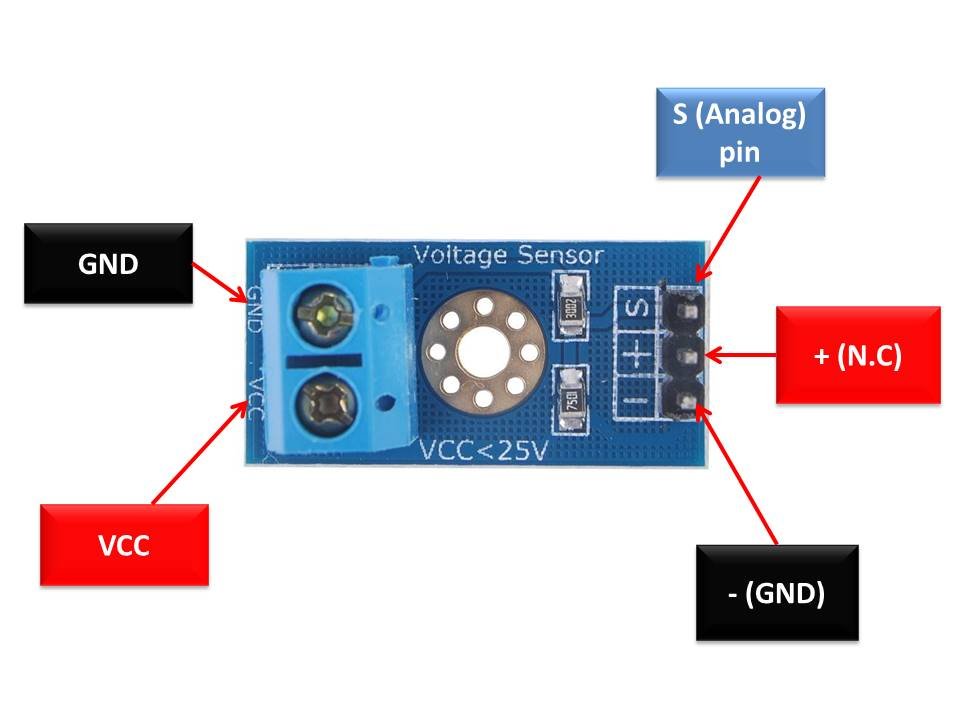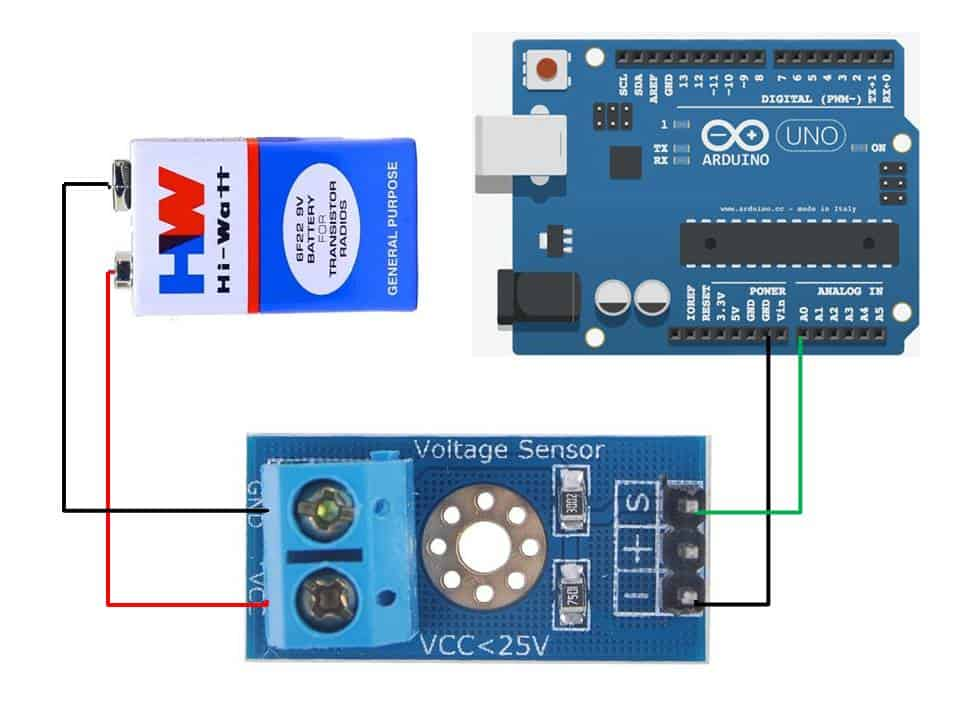1

### Description

The Voltage Sensor Module DC 0-25V is a small electronic module that can measure DC voltage in the range of 0-25V with an accuracy of ±0.5%. The module has three pins, VCC, GND, and OUT. It uses a voltage divider circuit to sense the voltage and provides an analog output signal that is proportional to the input voltage. The output voltage range is 0-5V, which can be interfaced with a microcontroller or an analog-to-digital converter (ADC). The module is commonly used in battery monitoring, DC motor control, and other low-voltage DC applications.

Package Includes:

• 1 x Voltage Sensor Module DC 0-25V

Features:

1. Voltage range: The module is designed to measure DC voltage in the range of 0-25V.
2. Accuracy: The module has an accuracy of ±0.5%.
3. Output signal: The module provides an analog output signal that is proportional to the input voltage. The output voltage range is 0-5V.
4. Sensing method: The module uses a voltage divider circuit to sense the voltage.
5. Power supply: The module operates on a DC voltage supply in the range of 3.3V to 5V.
6. Size: The module is small and compact.
7. Interface: The module has three pins, VCC, GND, and OUT, which can be connected to a microcontroller or an analog-to-digital converter (ADC).

Description:

The Voltage Sensor Module DC 0-25V is a small electronic module designed to measure DC voltage in the range of 0-25V with an accuracy of ±0.5%. The module has a voltage divider circuit that consists of two resistors. The input voltage is divided by the resistors to a level that can be measured by an analog input pin of a microcontroller or an ADC. The output voltage range of the module is 0-5V, which can be used with a microcontroller or an ADC that has a 10-bit analog-to-digital converter. The module has three pins, VCC, GND, and OUT, which can be connected to a microcontroller or an ADC. The VCC pin should be connected to a DC voltage supply in the range of 3.3V to 5V, and the GND pin should be connected to the ground of the circuit. The OUT pin provides an analog output signal that is proportional to the input voltage. The module also has a power LED that indicates when the module is powered on. The Voltage Sensor Module DC 0-25V is commonly used in battery monitoring, DC motor control, and other low-voltage DC applications where accurate voltage measurement is required. The module can be easily integrated into a circuit and can be used with a wide range of microcontrollers and ADCs. The small size of the module makes it ideal for use in applications where space is limited.

Principle of Work:

The module has a simple circuit design consisting of two resistors connected in series to form a voltage divider. The input voltage is applied across the two resistors, and the voltage across the second resistor is used as the output voltage. The output voltage is proportional to the input voltage and is calculated using the voltage divider formula:

Output voltage = (R2 / (R1 + R2)) x Input voltage

Where R1 and R2 are the resistances of the two resistors in the voltage divider circuit.

The module provides an analog output signal that is proportional to the input voltage. The output voltage range is typically 0-5V, which is suitable for use with microcontrollers or ADCs that have a 10-bit analog-to-digital converter. The module's simple circuit design and analog output make it easy to integrate with other electronics projects and systems that require DC voltage measurement.

Pinout of the Module:Pin Name Function
VCC Positive power supply pin. The positive terminal of the power supply to be measured is connected to this pin.
GND Reference potential pin. It is connected to the negative terminal of the power supply.
S Analog output pin voltage of the sensor. Connect it to the analog input pin of Arduino or any other microcontroller which you want to use.
+ Not connected
Ground connection pin. It must be joined to the ground pin of the Arduino or microcontroller and also to the power supply ground pin.

Applications:

1. Battery monitoring: The module can be used to monitor the voltage of a battery in real time, providing a useful tool for measuring the state of charge and predicting battery life.
2. Power supply monitoring: The module can be used to monitor the output voltage of a power supply, ensuring that it is operating within its specified range.
3. Robotics: The module can be used in robotics projects to monitor the voltage of motors and other components, helping to prevent damage and prolong the life of the components.
4. Home automation: The module can be used in home automation systems to monitor the voltage of various devices, such as lights and appliances, allowing for more efficient and intelligent control.
5. Industrial automation: The module can be used in industrial automation systems to monitor the voltage of machinery and equipment, ensuring that they are operating within safe and optimal ranges.

Circuit:

This circuit will monitor the 9V battery using Arduino on pin A0:Library:

No library Needed

Code:

code for monitoring the voltage of a 9V battery using the Voltage Sensor Module DC 0-25V and Arduino:

```int sensorPin = A0;
float voltage;
float threshold = 7.5; // Threshold voltage for low battery warning
bool isLow = false; // Flag for low battery status

void setup() {
Serial.begin(9600);
}

void loop() {

// Convert the sensor value to voltage
voltage = sensorValue * (5.0 / 1023.0) * 5.0;

// Print the voltage value to the serial monitor
Serial.print("Voltage: ");
Serial.print(voltage);
Serial.println("V");

// Check if the voltage is below the threshold and set the low battery flag
if (voltage < threshold && !isLow) {
isLow = true;
Serial.println("Low battery warning!");
}
else if (voltage >= threshold && isLow) {
isLow = false;
Serial.println("Battery is OK now.");
}

delay(1000);
}

```

This code reads the analog voltage value from the module on pin A0, converts it to voltage, and prints it to the serial monitor every second. It also checks if the voltage is below a certain threshold (in this case, 7.5V), and if so, sets a flag and prints a low battery warning to the serial monitor. When the voltage is above the threshold again, it resets the flag and prints a message indicating that the battery is OK.

You can interact with the program by changing the `threshold` value to set your own low battery warning threshold, or by changing the `delay` value to adjust how frequently the voltage value is read and printed to the serial monitor. You can also add more interactive features, such as setting an LED to turn on when the battery is low or sending a notification to your phone using a Wi-Fi module.

Technical Specifications:

• Input Voltage Range: DC 0-25V
• Output Voltage Range: DC 0-3.3V
• High Accuracy: 1%
• On-board Voltage Divider: Can be used to divide input voltage if required
• Small size and easy to use
• Analog output signal
• Operating Voltage: 5V DC
• Operating Current: <10mA
• Size: 4cm x 3cm x 2cm
• Weight: 2g

Resources:

Comparisons:

The ZMPT101B module and the Voltage Sensor Module DC 0-25V are two different types of voltage sensing modules designed for different applications. Here's a comparison of their features:

1. Voltage range: The ZMPT101B module is designed to measure AC voltage in the range of 0-250V, while the Voltage Sensor Module DC 0-25V is designed to measure DC voltage in the range of 0-25V.
2. Output signal: The ZMPT101B module provides an analog voltage output signal in the range of 0-5V from 220vAC, while the Voltage Sensor Module DC 0-25V provides an analog output signal that can be interfaced with a microcontroller only 5 times smaller than the original signal.
3. Sensing method: The ZMPT101B module uses a voltage transformer and an operational amplifier circuit to sense the voltage, while the Voltage Sensor Module DC 0-25V uses a voltage divider circuit.
4. Accuracy: The ZMPT101B module is more accurate than the Voltage Sensor Module DC 0-25V because it compensates for the non-linearities and other factors affecting the voltage measurement.
5. Application: The ZMPT101B module is commonly used in power monitoring, home automation, industrial control systems, voltage regulators, and power supplies, while the Voltage Sensor Module DC 0-25V is commonly used in battery monitoring, DC motor control, and other low voltage DC applications.